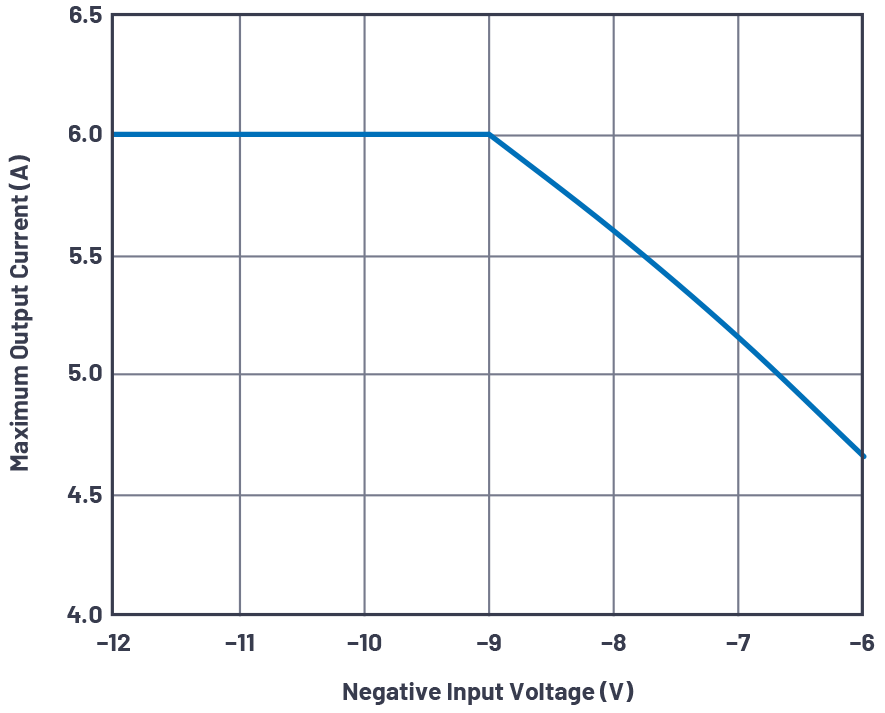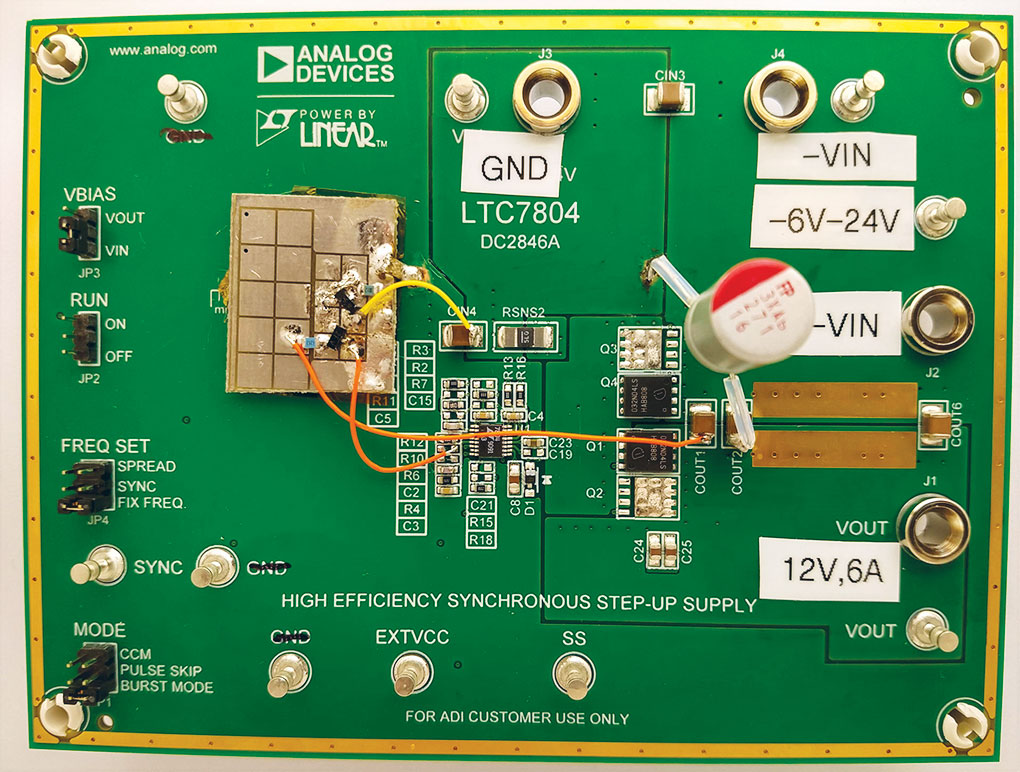Registration Required

The username/email you selected is already registered. Please choose a different one. This email domain is blocked. Please use different domain Blocked domain

Send new code

1. 8-16 characters
2. Containing 3 out of 4 of the following:
• Lowercase characters
• Uppercase characters
• Digits (0-9)
• One or more of the following symbols:
@ # \$ % ^ & * - _ + = [ ] { } | \ : ' , ? / ` ~ " ( ) ; .

# Add an Efficient Positive Rail to a Unipolar Negative Supply

### Introduction

Sometimes you need a positive power supply, and the most available rail (or only available rail) is negative. In fact, negative-to-positive voltage conversion is used in automotive electronics, and the biasing circuitry for a variety of audio amplifiers, and industrial and test equipment. Even though power is distributed in many of these systems via a negative—relative to ground—rail, the logic boards, ADCs, DACs, sensors, and similar devices found in them still require one or more positive rails. This article presents a simple, low component count, efficient circuit for the generation of a positive voltage from a negative rail.

### Circuit Description and Power Train Functionality

Figure 1 shows a complete solution for efficient conversion of a negative voltage to a positive voltage. This particular solution uses a boost topology. The power train includes switching MOSFETs, bottom Q1, top Q2, inductor L1, and input/output filters. The synchronous high efficiency boost controller IC regulates output voltage by changing the state of the switching MOSFETs in the power train. For the purposes of describing this circuit, system ground (SYS_GND) serves as the reference regarding polarity, with a negative—relative to SYS_GND—input rail (–VIN), and positive—relative to SYS_GND—output rail (+VOUT).

The converter works as follows. If transistor Q1 is on, then current flows from the SYS_GND to the negative rail. The transistor Q2 is off and inductor L1 stores energy in its magnetic field. To complete the switching period, Q1 turns off, and Q2 turns on. Current starts to flow from the SYS_GND to the +VOUT rail, discharging L1 energy to the load.Figure 1. A negative-to-positive converter electrical schematic, with VIN –6 V to –18 V (–24 V peak) and with VOUT +12 V at 6 A.

### Basic Expressions for the Power Train Components Selection

The topological diagrams in Figure 2 of the switching behavior illustrate the negative-to-positive converter behavior. For the first interval of a switching cycle, over a length of time defined by the duty cycle, the bottom switch, BSW, is shorted and the top switch, TSW, is open. The voltage across the inductor, L, is equal to –VIN. Throughout this interval, current in inductor L increases, generating a voltage polarity matching –VIN across the inductor. At the same time, the output filter capacitor discharges, supplying current to the system load.

The second interval of the cycle flips both switches—BSW is open and TSW is shorted. The polarity across inductor L changes, and the inductor starts sourcing current (stored in the first interval of the cycle) to both the load and COUT, the output filter capacitor. The inductor sees a corresponding decrease in current over this part of the cycle. The volt-second balance of the inductor defines the duty cycle, D, of the converter in continuous conduction mode.

### Calculating Timing and Component Stresses

Here are the formulas describing the timing and stresses of the power train components.

The duty cycle determines the on/off time of the switches

The average value of the input current, IOUT, is input current

The peak value of the inductor current

The voltage stress on the switching MOSFET

The average current through the bottom MOSFET

The average current through the top MOSFET

These expressions are useful for a general understanding of the functionality of the topology and for preliminary selection of the power train components. For final selection and detailed design, please use LTspice® modeling and simulation.1

### Converter Control Description and Functionality

Sensing of the output voltage and level shifting of the control voltage are managed by the current mirror based on the PNP transistors Q3 and Q4. The feedback current IFB (1 mA in this circuit) determines the value of the resistors in the feedback loop.

where VC is the reference voltage of error amplifier.

where RFB(T) is the output voltage sensing resistor.

The feedback circuit presented in Figure 1 is an inexpensive solution, but tolerance of discrete transistors can be affected by the differences in base emitter voltage and temperature variations. To improve accuracy, a matched pair transistor can be used.

Control of the converter power train is left to the LTC7804 boost controller. This chip was selected due to its high efficiency by means of synchronous rectification, easy implementation, high switching frequency operation (if a small inductor size is desired), and low quiescent current.

### Test Results and Topology Limitations

This solution was meticulously tested and verified. Figure 3 shows that efficiency remains high over a wide range of load currents—reaching 96%. Note that as the absolute value of the input voltage decreases, the input and inductor current increases. At a certain point, the inductor current can exceed the maximum, or saturation current, on the inductor. The derating curve showing this effect is shown in Figure 4. The maximum load current is 6 A in the range from –9 V to –18 V, falling below that for input voltages with absolute values below –9 V. Thermal performance is shown in Figure 5 for the solution board in Figure 6.Figure 3. Efficiency curve for VIN –12 V and –18 V with natural convection cooling.Figure 4. Output current derating curve for absolute value input voltages below –9 V.Figure 5. Thermal image of the converter with VIN –12 V and VOUT +12 V at 6 A, using natural convection cooling with no air flow.Figure 6. Converter photo.

### Conclusion

This article presents a complete solution for a very efficient and relatively simple design for adding a positive rail to a unipolar negative power supply using a boost controller. It also provides electrical schematics and calculations for timing, power conversion components, and electrical stress. Test data confirms high efficiency and good thermal performance. Furthermore, the boost topology used in this solution gives the designer the option to use a prequalified boost controller, saving development time and cost. Conversely, qualifying a boost controller for a negative-to-positive converter can prequalify it for future boost applications.